# Introduction#

A binary heap is a heap data structure that uses a binary tree to store items.

To use a binary tree as a binary heap, it needs to sort the items it stores. The binary heap can store the items in descending order, as a max heap, or in ascending order, as a min heap.

To implement a binary tree as a max heap, every parent node must be greater than or equal to its children.

To implement a binary tree as a min heap, every child must be greater or equal than their parent.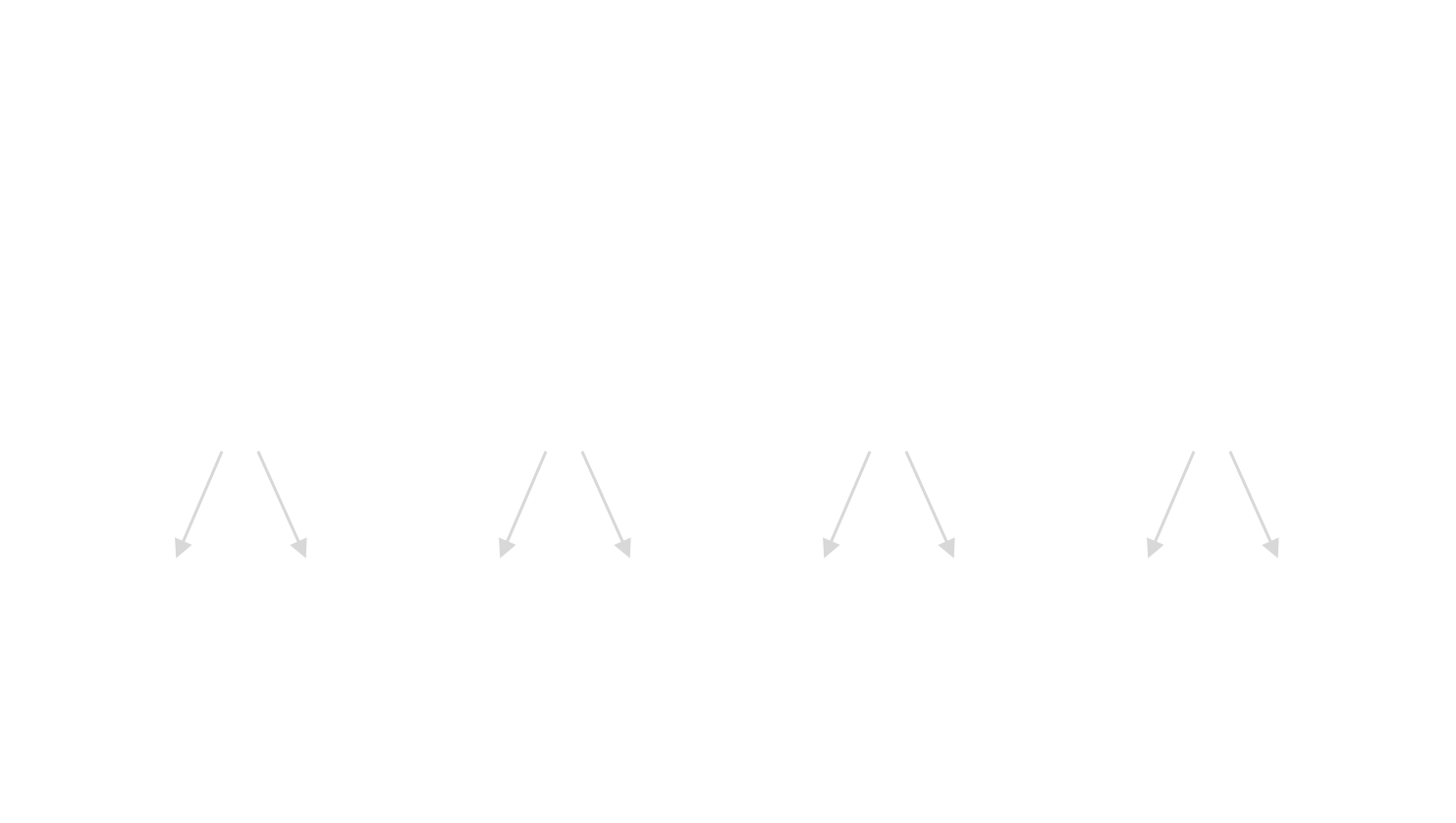A Min Heap. The node at the root of the tree has the smallest value. As the depth of the tree increases, the values will increase as the value of the child node is always greater (or equal) when compared to the parent node.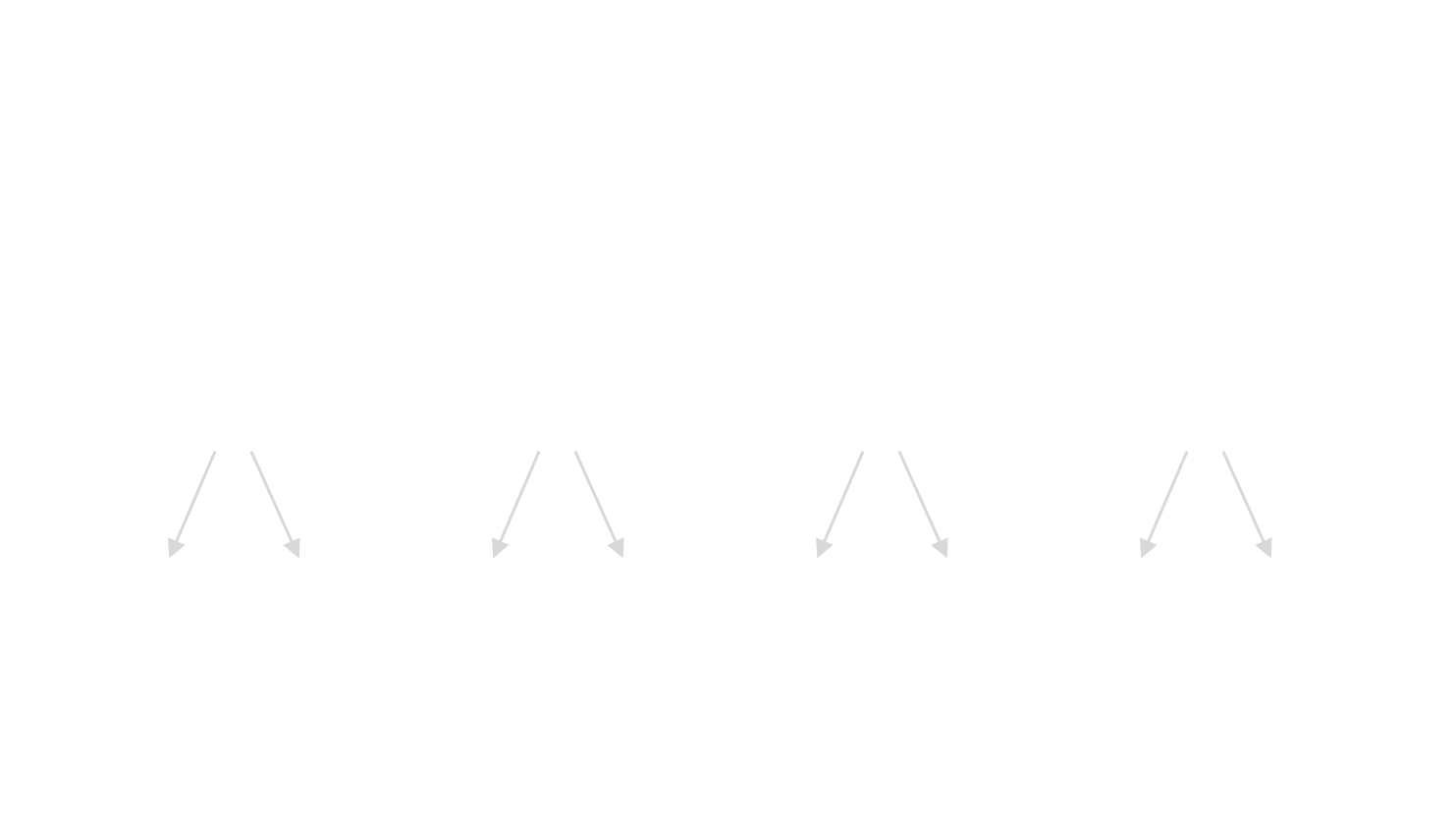A Max Heap. The node at the root of the tree has the greatest value. As the depth of the tree increases, the values will decrease as the value of the child node is always lesser (or equal?) when compared to the parent node.

A binary heap is often used to implement a priority queue, which is another data structure. Those two are so similar that binary heaps are sometimes referred as a priority queue and vice-versa; the main difference between those is the names of the method they expose.

# Implementation#

### A binary heap is an array#

It is recommended to implement a binary heap using a dynamic array because it perfectly represents a binary tree.

To provide some background about this statement, let’s pick an array with three items, for example, [10, 20, 30].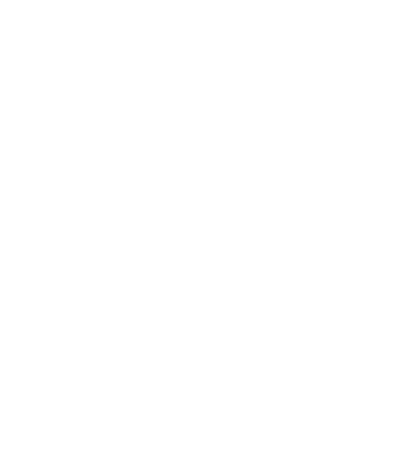The first item of the array is the root of the binary tree (10). Its children’s index positions in the array will be at the N*2+1 for the left child (20) and N*2+2 for the right child (30) where N is the parent position.

Thanks to the formula used to get the left and right children’s index of a node, it is possible to determine that all the left nodes of a binary tree are in an odd position, and on the other side, all the right nodes are in an even one.

The second item in the example array (20) is the left child of the first item (10), and it can find the parent node index at the N/2 position, where N is the child position.

The third and last item in the example array (30) is the right child of the first item (10); similarly to the left node, it can find the parent index of a right node at the N/2-1, where N is the child position.

Note: all the images have the binary tree representation at the top and the binary representation at the bottom

### Go implementation#

The binary heap implementation discussed in this article is a type BinaryHeap which is a max heap. At the end there are some notes on how to use the approach for a min heap as well.

The only difference between the max heap and the min heap is about how the nodes are compared. Where N1 is the parent of N2, in a MaxHeap N1 > N2, but in a MinHeap will be N1 < N2.

The BinaryHeap type has the following fields:

• items: A slice of integer, where the binary heap will store its items; for more complex implementations, it is recommended to use a custom type instead of an integer.
• mutex: a mutex to ensure that the binary heap is thread-safe.

In Go, an implementation may look like this:

``````type BinaryHeap struct {
items []int
mu    sync.Mutex
}
``````

### Insert#

Inserting a new node in a binary heap is interesting and not very complex. It just involves a bit of logic.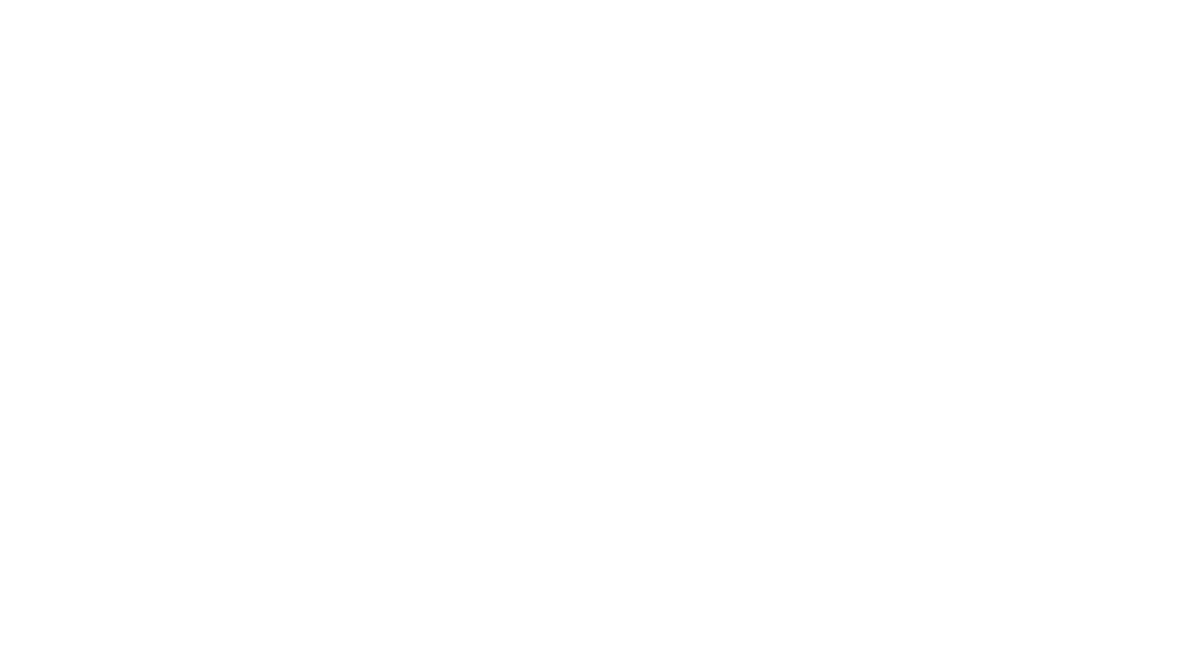Few nodes need to be added to a binary heap with four nodes. New nodes are always placed at the end of the binary heap, starting at the leftmost open space of the tree's bottom level.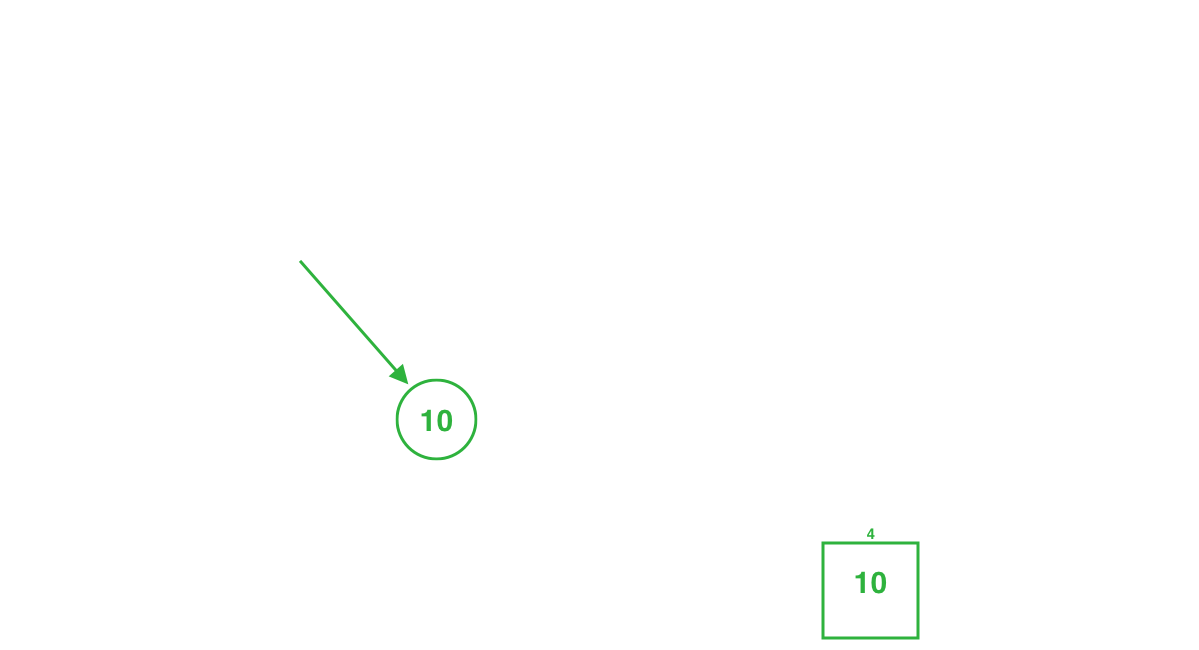Case 1: A node (10) has been added to the max heap. Since it is smaller than its parent (20), it is at the correct position, and there are no more operations to perform.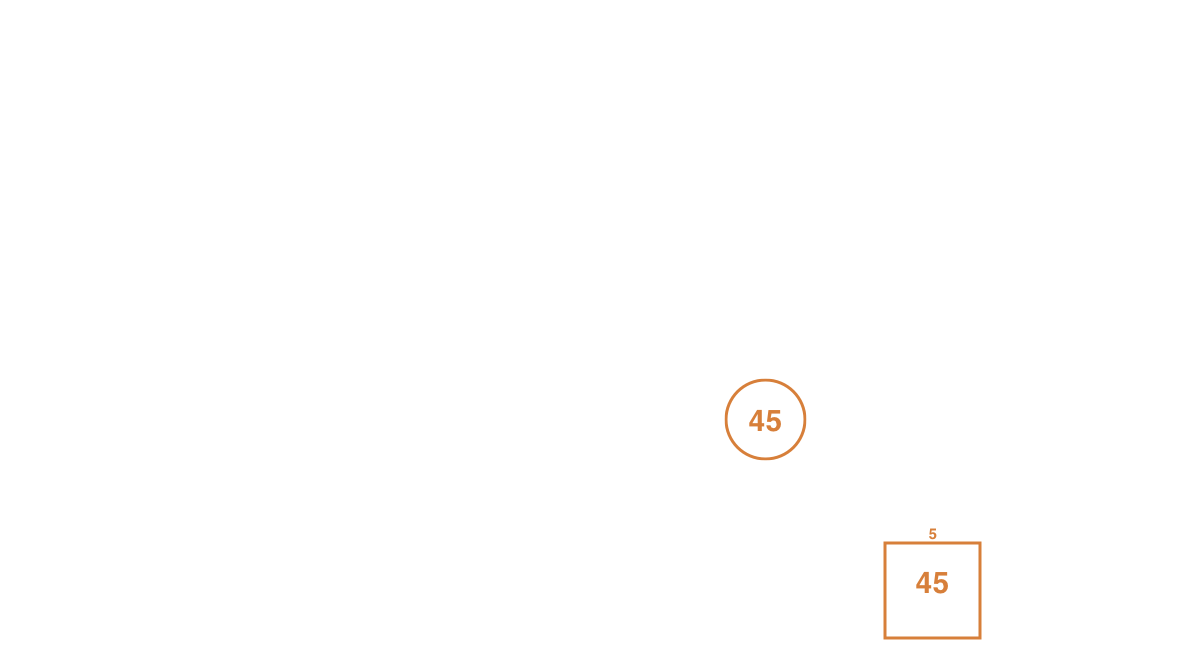Case 2: A node (45) is added but its parent (15) has a smaller value. Thus, the new node needs to sift up to keep the heap sort.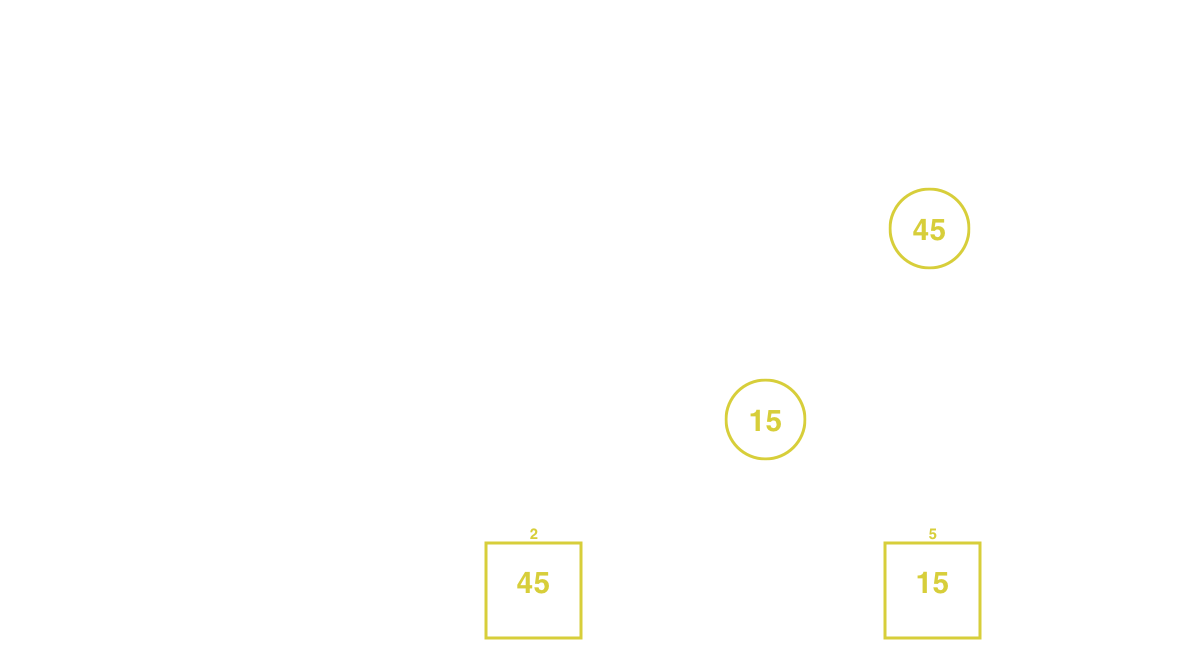Sifting up entails swapping the new node with its parent node to guarantee that there will be a smaller value in the lower position.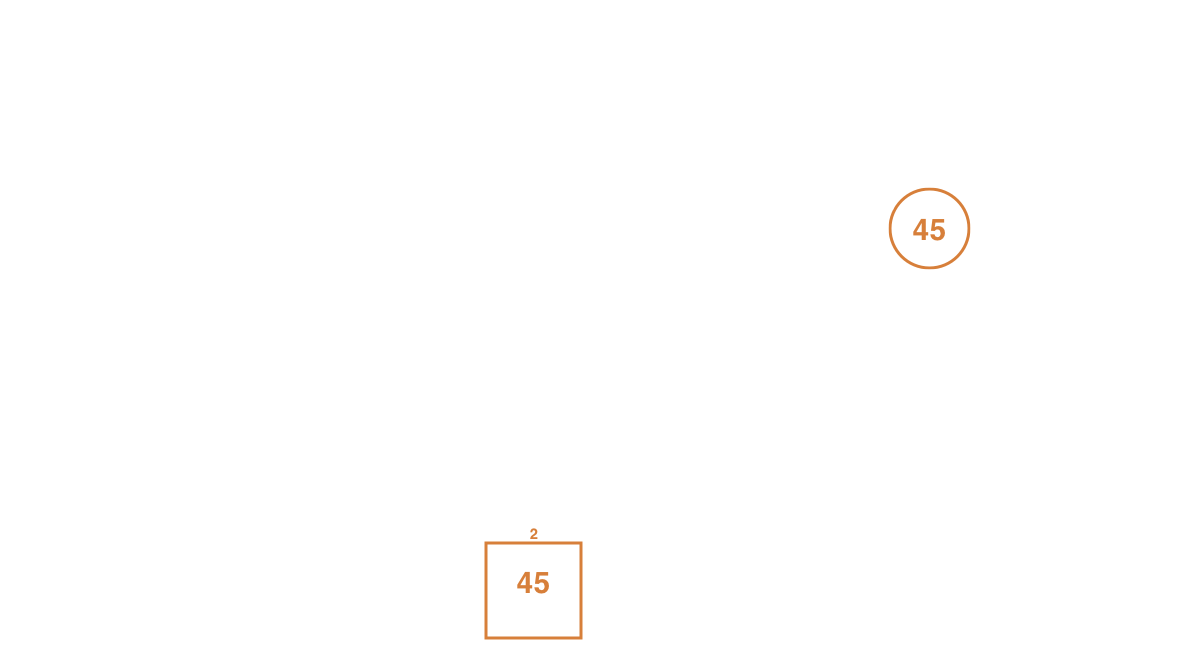In this case, sifting up once is not enough as the max heap is still not valid (45 > 30). The node just sifted up (45) needs to continue to sift up until its parent is greater or equal.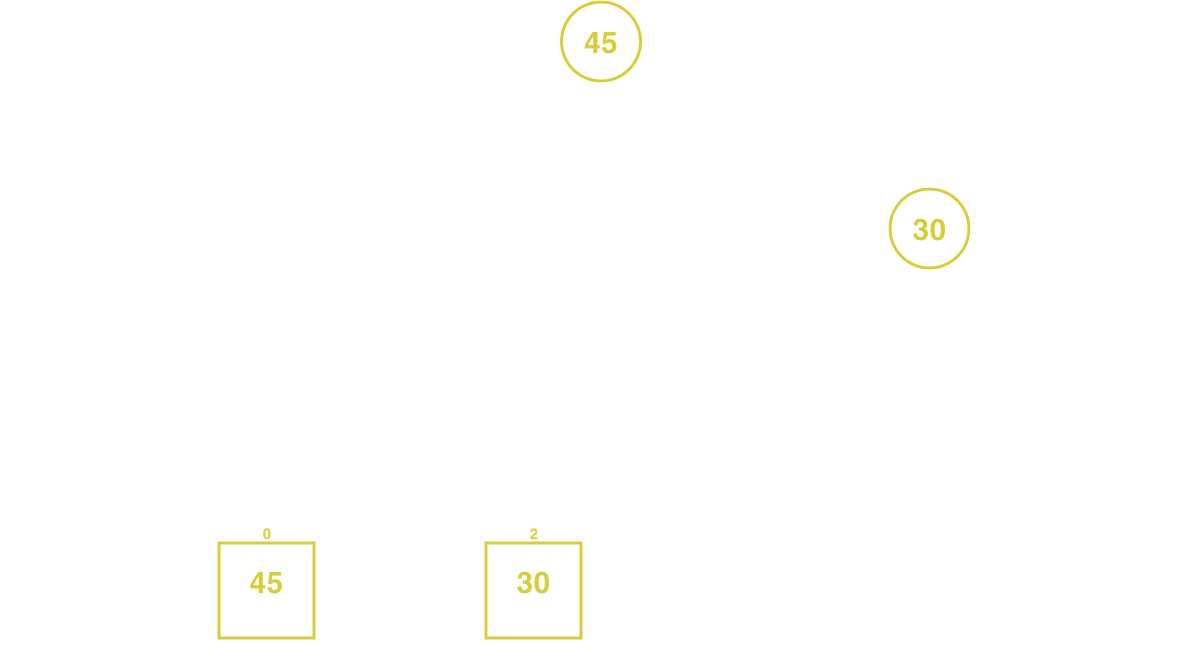Once more, swap the node (45) with its parent (30). Now that the node as reached the root, the binary heap is sorted.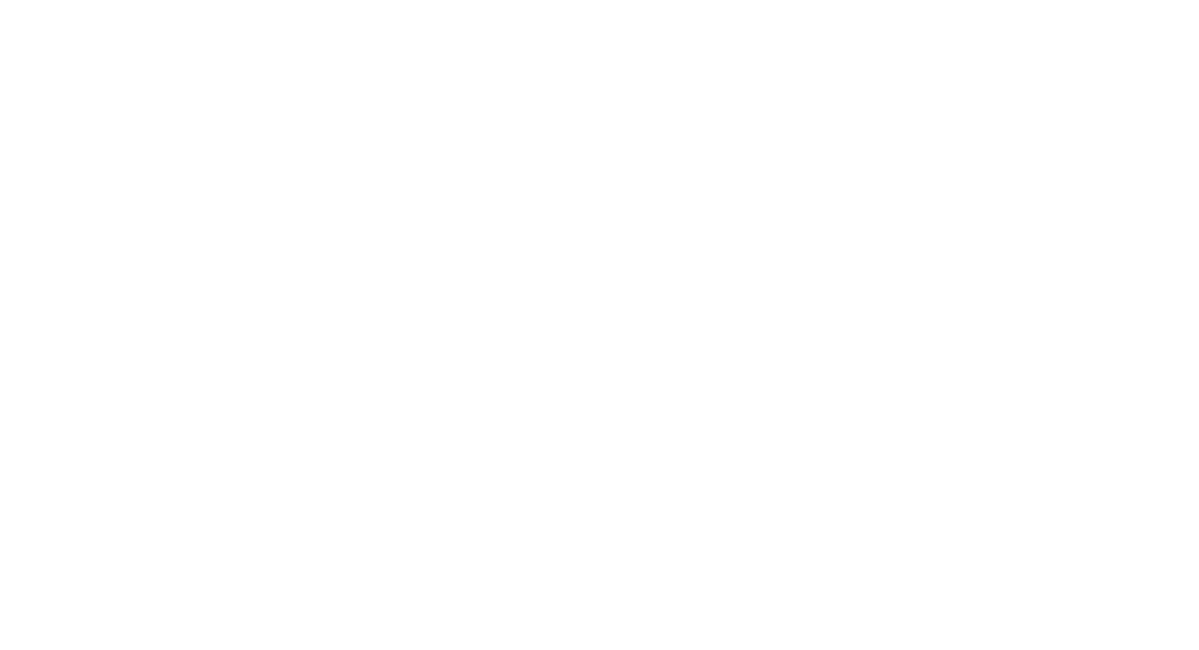The complexity to add a node in a binary heap is an O(log n). For a min heap, it is possible to insert a node using the same approach but sifting up when a parent node is greater than the inserted one.

An implementation in Go may look like this:

``````func (h *BinaryHeap) Insert(v int) {
// lock meanwhile the operation is ongoing
h.mu.Lock()
defer h.mu.Unlock()

// add the new item and sift up to have it placed at the correct position
h.items = append(h.items, v)
h.siftUp(len(h.items) - 1)
}

func (h *BinaryHeap) siftUp(idx int) {
for {
// get the parent index
parentIdx := h.parent(idx)
// if the parent value is greater or equal than the current index, the heap is sorted and there is no need to keep sorting
if h.items[parentIdx] >= h.items[idx] {
return
}
// switch the parent index with the current index
h.items[parentIdx], h.items[idx] = h.items[idx], h.items[parentIdx]
// update the current index and continue sifting up the node
idx = parentIdx
}
}

func (h *BinaryHeap) parent(i int) int {
if i%2 == 0 {
i--
}
return i / 2
}
``````

### Extract#

Extracting a node from a binary heap is an operation which will always return the greatest value in case of a max heap, and the smallest one in case of a min heap.

After an extraction happens, the binary heap needs to guarantee the order of the items for the next insertion or extraction.Example: Extract nodes from a binary heap with six items.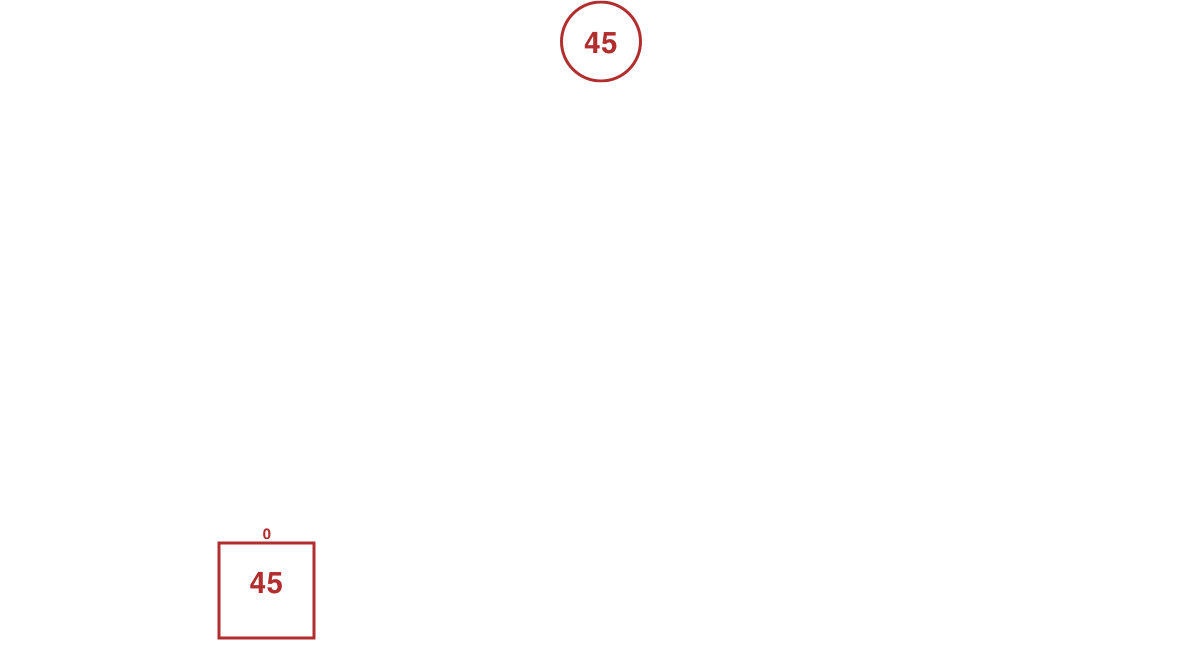This is a max heap so the largest value, the root node (45), is the node that will be extracted.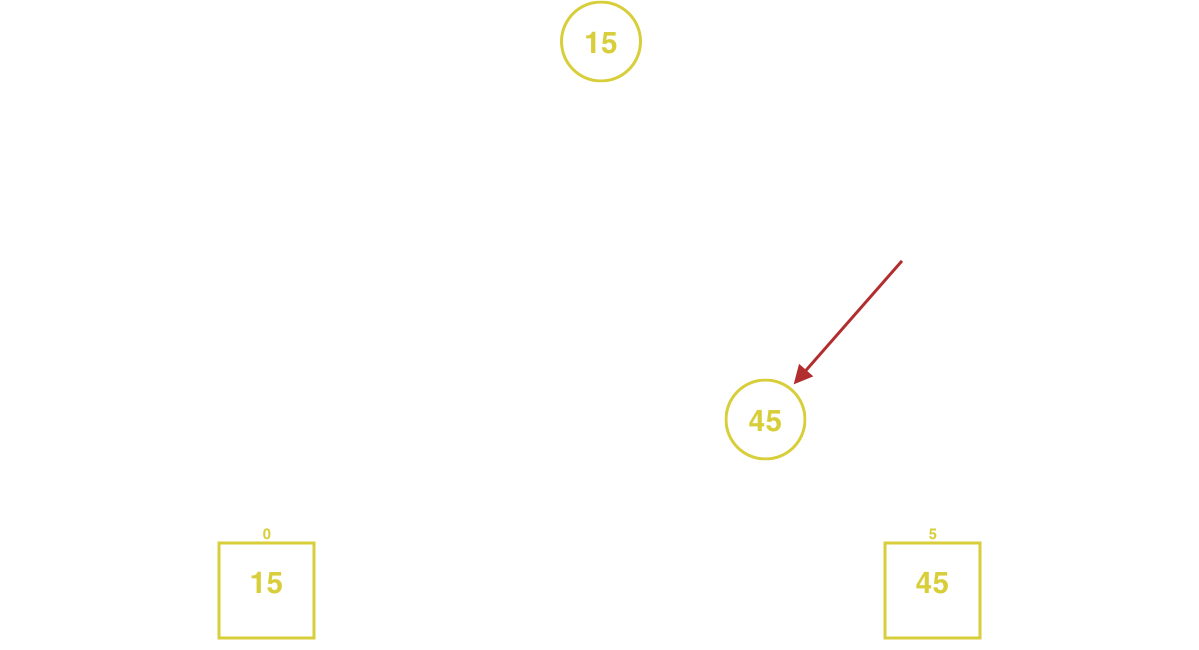The root node (45) is swapped with the most recently added node (15) so that it can safely be extracted without leaving orphan nodes.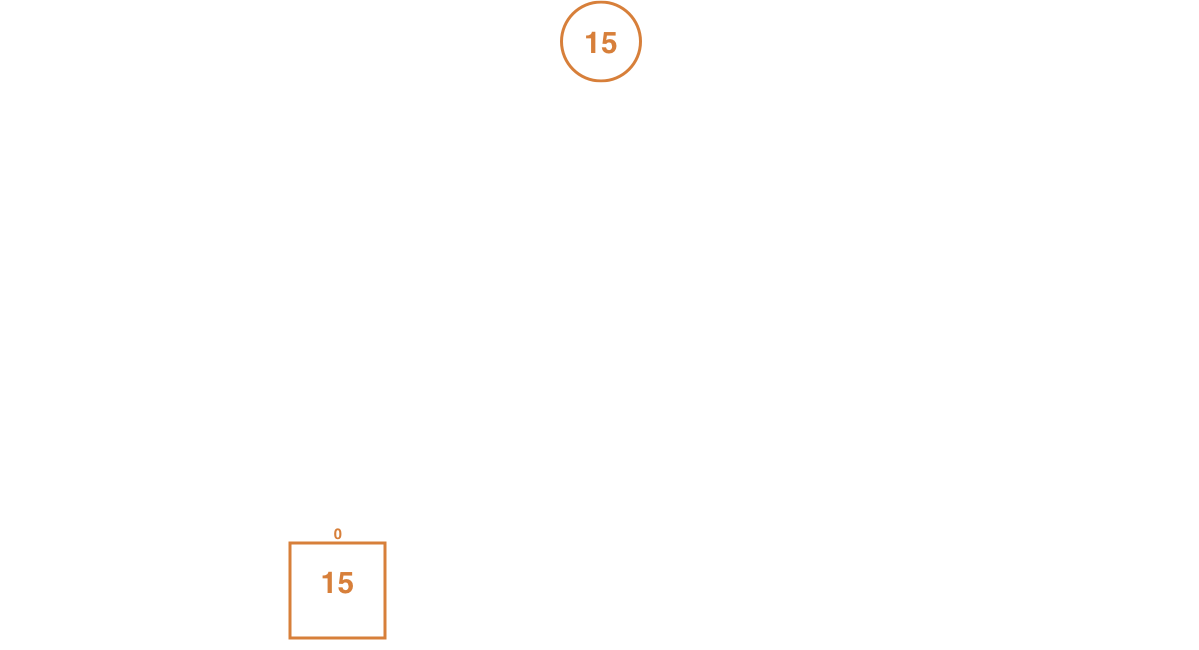The binary heap is now left in a state which doesn't guarantee the order of the items. Extracting another item at this state won't return the node with the greatest value.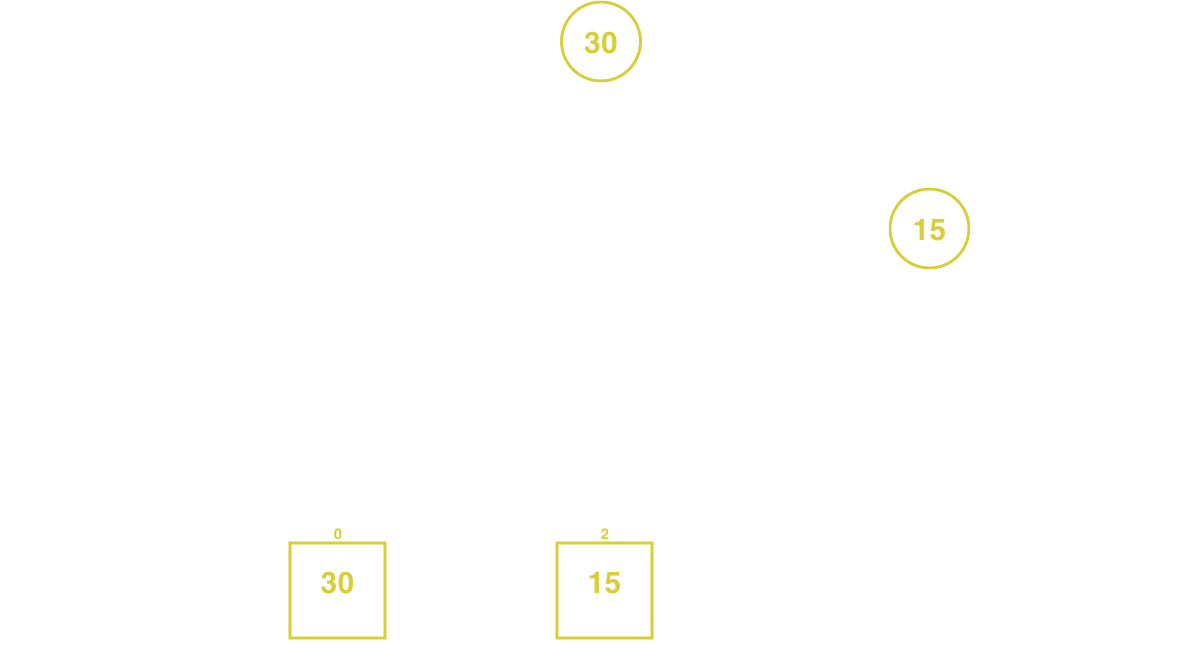The current root node (15) need to be sift down with its greatest child (30). This operation is repeated until the node does not have children with a greater value than itself.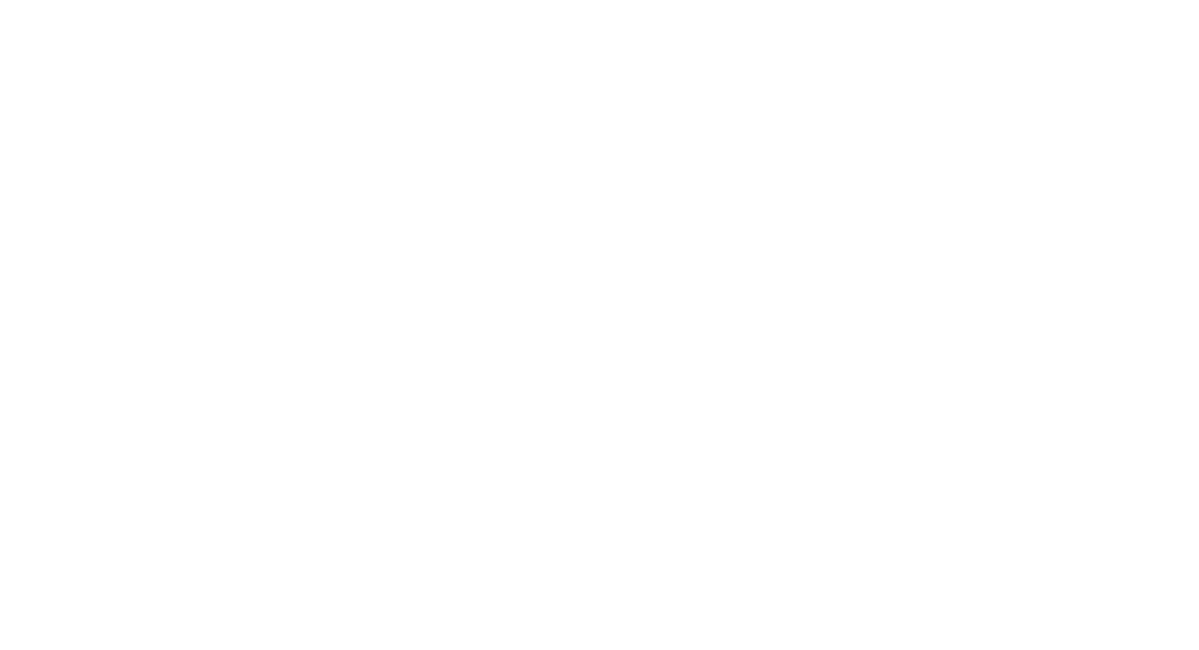The complexity to extract a node in a binary heap is an O(log n). It is possible to extract a node from a min heap using the same approach, with the only difference as sifting down when the swapped node has a smaller child node.

The extract method can be implemented in this way:

``````func (h *BinaryHeap) Extract() (int, bool) {
// lock meanwhile the operation is ongoing
h.mu.Lock()
defer h.mu.Unlock()

// if there are no items
// return false to notify that the operation did not extract any element from the binary heap
if len(h.items) == 0 {
return 0, false
}

// select min and max indexes from the heap
minIdx, maxIdx := 0, len(h.items)-1

// assign the root value to a variable to return once the root node is dropped
v := h.items[minIdx]
// switch min and max position, then remove the last item
h.items[minIdx], h.items = h.items[maxIdx], h.items[:maxIdx]

// sift down the new root to have it placed at the correct position
h.siftDown(minIdx)
return v, true
}

func (h *BinaryHeap) siftDown(idx int) {
for {
// get the right and left index from the current one
switch rIdx, lIdx := h.right(idx), h.left(idx); {

// in case the right node doesn't exist in the tree
case rIdx >= len(h.items):
// if the left node exists and is greater than the current node swap it
if lIdx < len(h.items) && h.items[lIdx] > h.items[idx] {
h.items[idx], h.items[lIdx] = h.items[lIdx], h.items[idx]
}
// stop because the current node has smaller children
return

// in case the left node doesn't exist in the tree
case lIdx >= len(h.items):
// if the right node exists and is greater than the current node swap it
if rIdx < len(h.items) && h.items[rIdx] > h.items[idx] {
h.items[idx], h.items[rIdx] = h.items[rIdx], h.items[idx]
}
// stop because the current node has smaller children
return

// in case the current node is greater than both left and right nodes
// stop because the binary heap is sorted
case h.items[idx] > h.items[rIdx] && h.items[idx] > h.items[lIdx]:
return

// in case the left is greater than the right one
case h.items[rIdx] < h.items[lIdx]:
// swap the left with the current node
// update the index to the left index and continue sorting
h.items[idx], h.items[lIdx] = h.items[lIdx], h.items[idx]
idx = lIdx

// in case the right if greater than the left one
case h.items[rIdx] >= h.items[lIdx]:
// swap the right with the current node
// update the index to the right index and continue sorting
h.items[idx], h.items[rIdx] = h.items[rIdx], h.items[idx]
idx = rIdx

// in case none matched stop
default:
return
}
}
}

func (h *BinaryHeap) left(i int) int {
return i*2 + 1
}

func (h *BinaryHeap) right(i int) int {
return i*2 + 2
}
``````

### Heapify#

Heapify is an operation that creates a binary heap starting from an unsorted array.

To heapify an array, it is needed to sift down each element starting from the last entry in the array to the first one.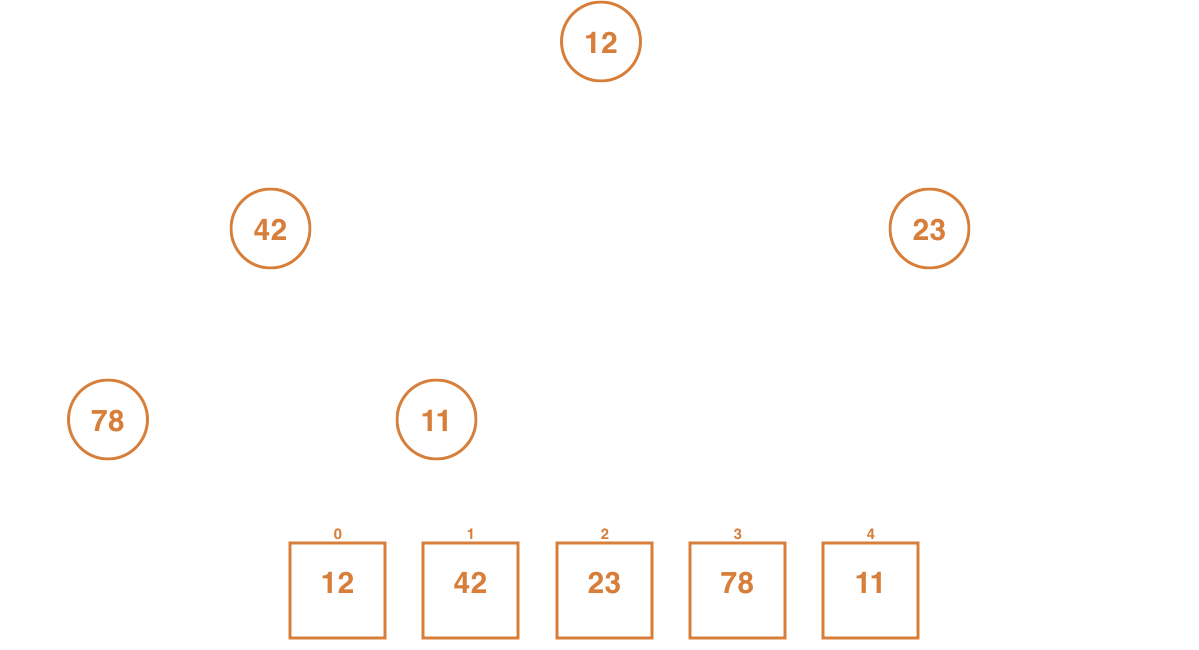An array with five nodes needs to be transformed into a max heap.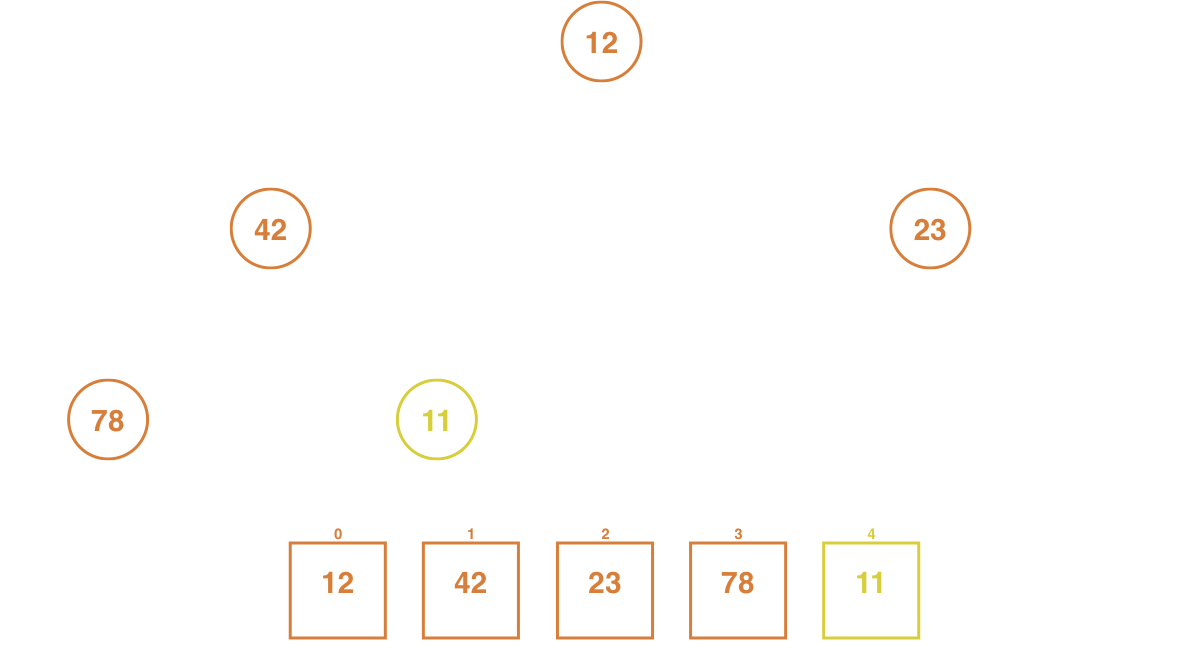To heapify the array, start from the last item to the first. The last one in this array is the node with a value of 11.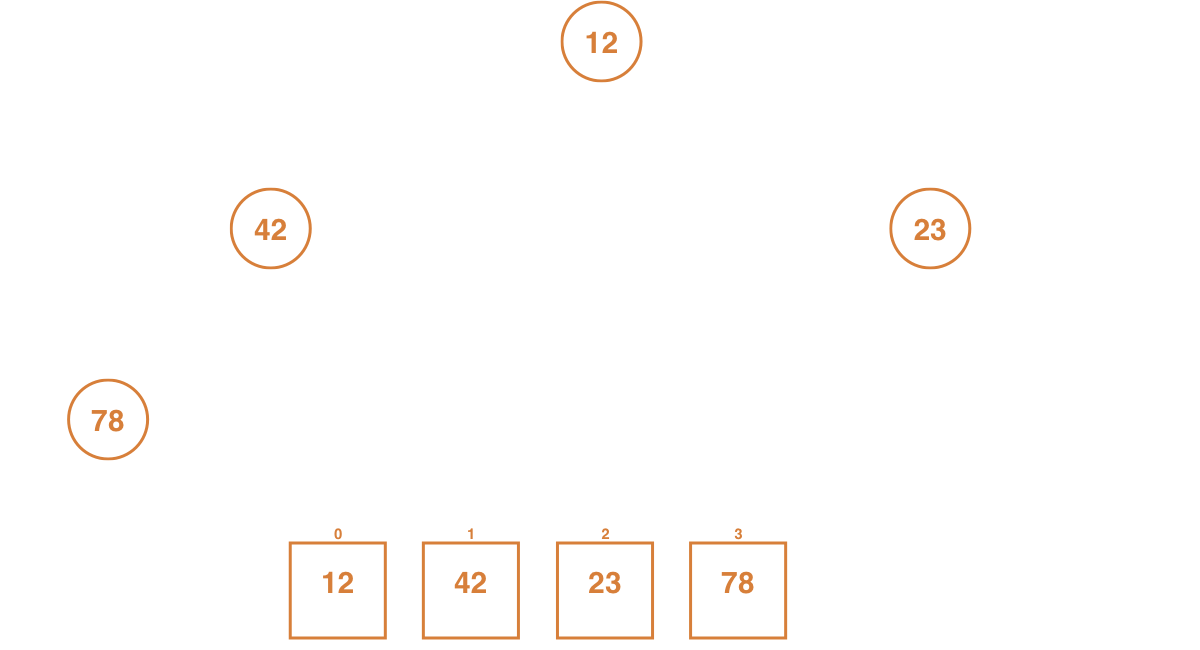Once selected, check if it has children. Being the last node, it will not have any.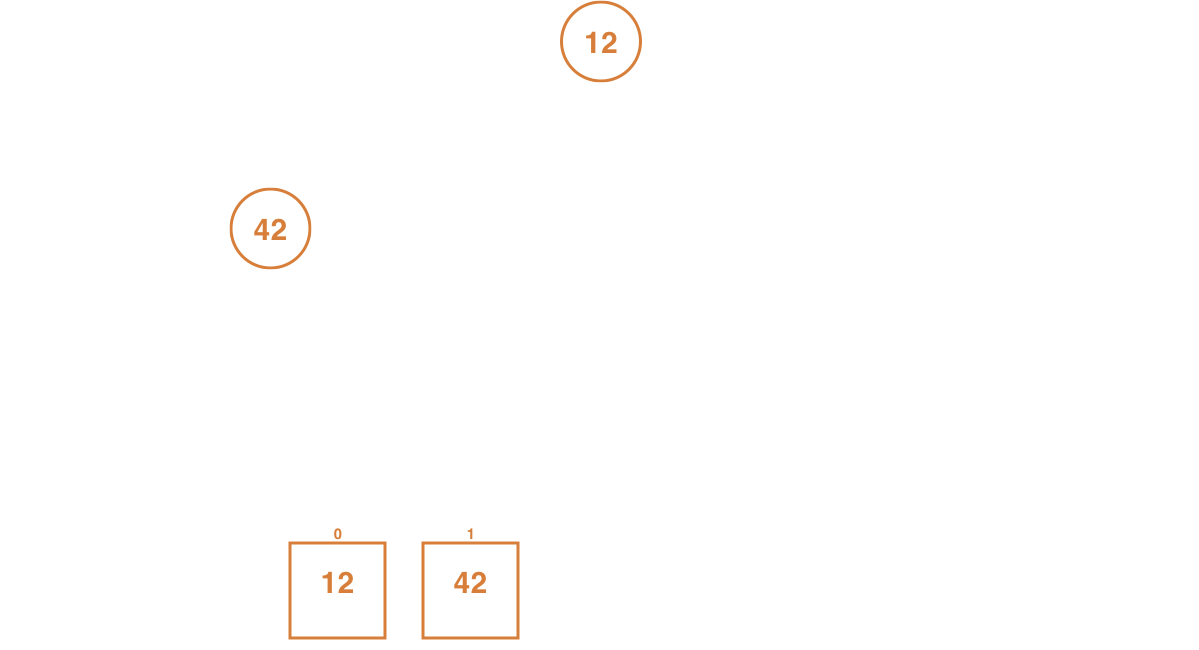Keep iterating through the array until a node with a child is encountered, which is the second node (42).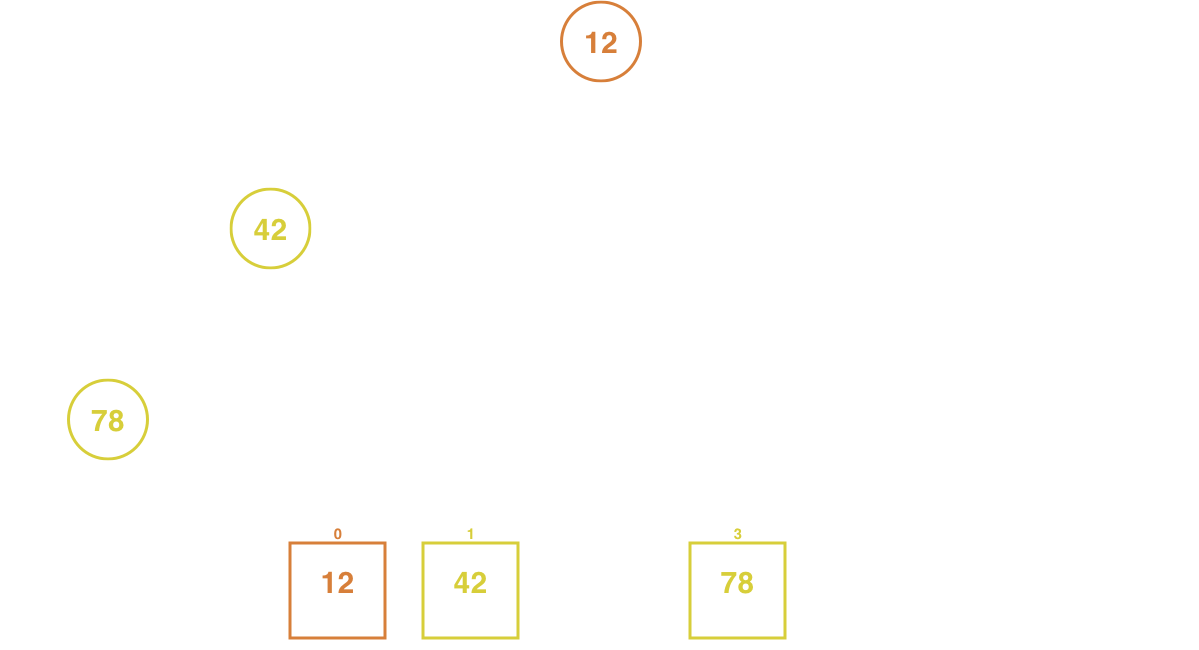The second node (42) has two children. If one of them is greater (78) than the current node (42), swap the position by sifting down.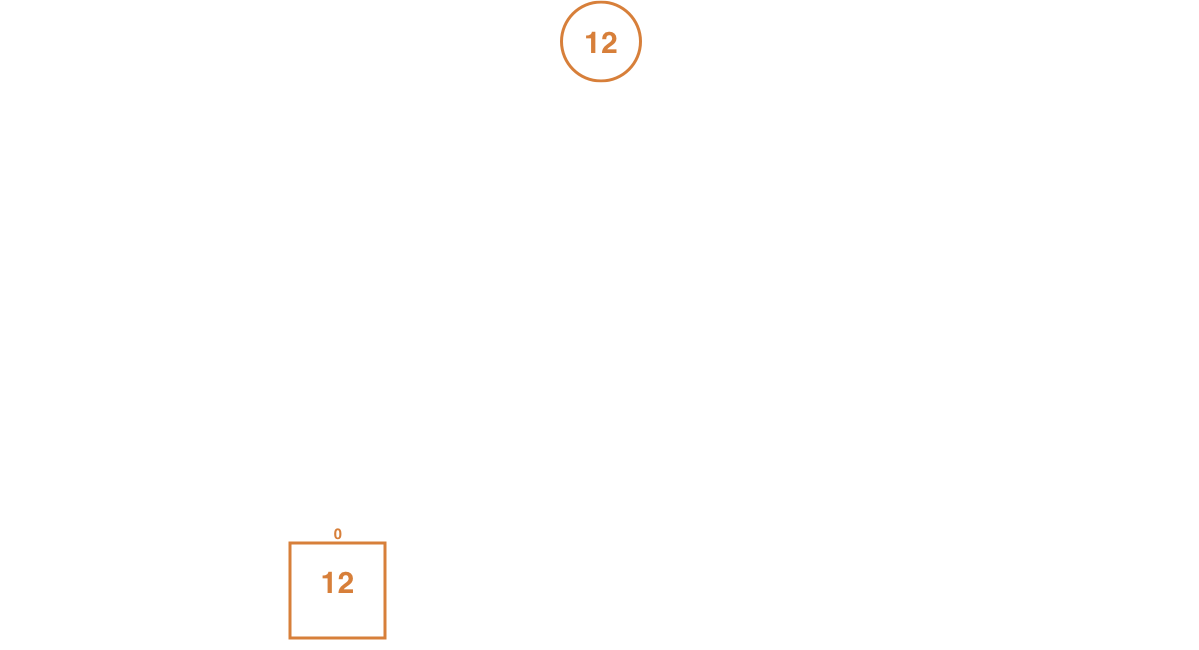The value of the second node (78) and the forth node (42) have been swapped. This is a sift down.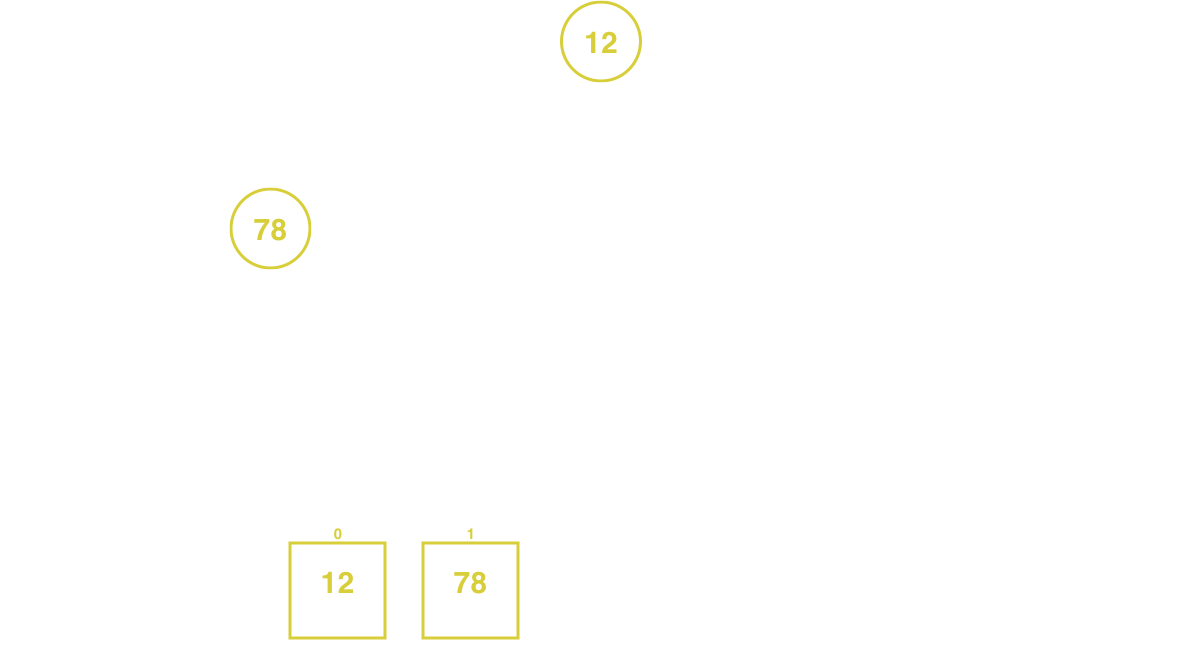The last node in the array with children is the root node (12). Both of the root node's children has a value greater than itself. In this case, select the child node with the larger value (78).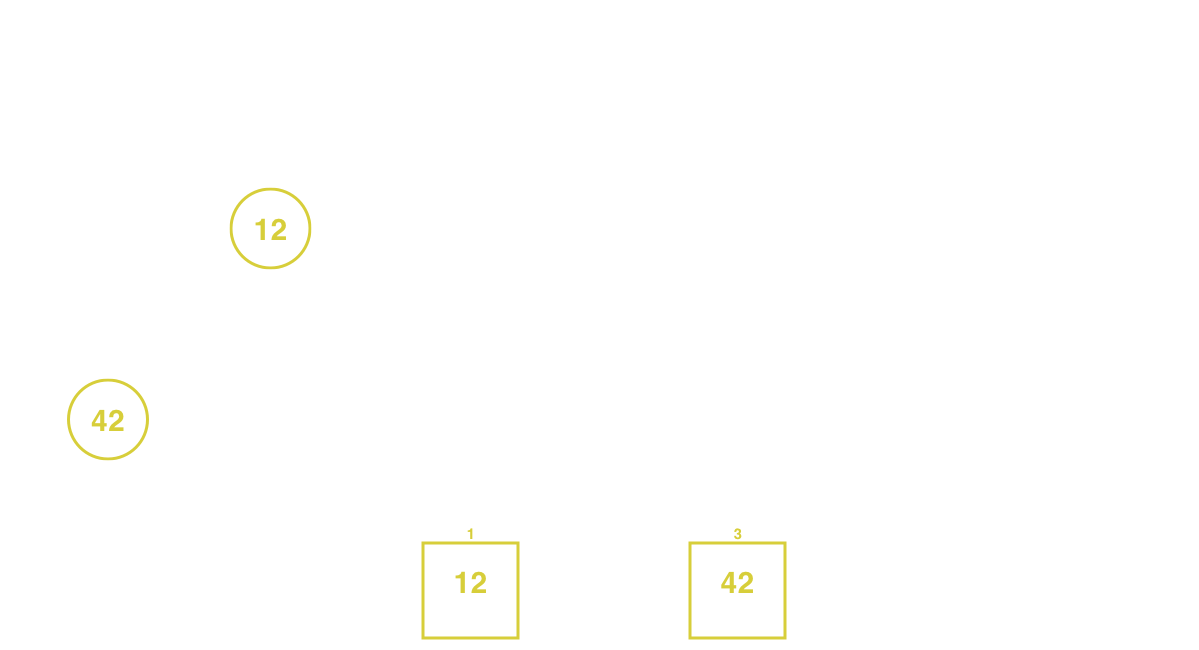Sift down the position and keep sifting until the node is greater than its children. Once all the nodes have been traversed, the array is a binary heap.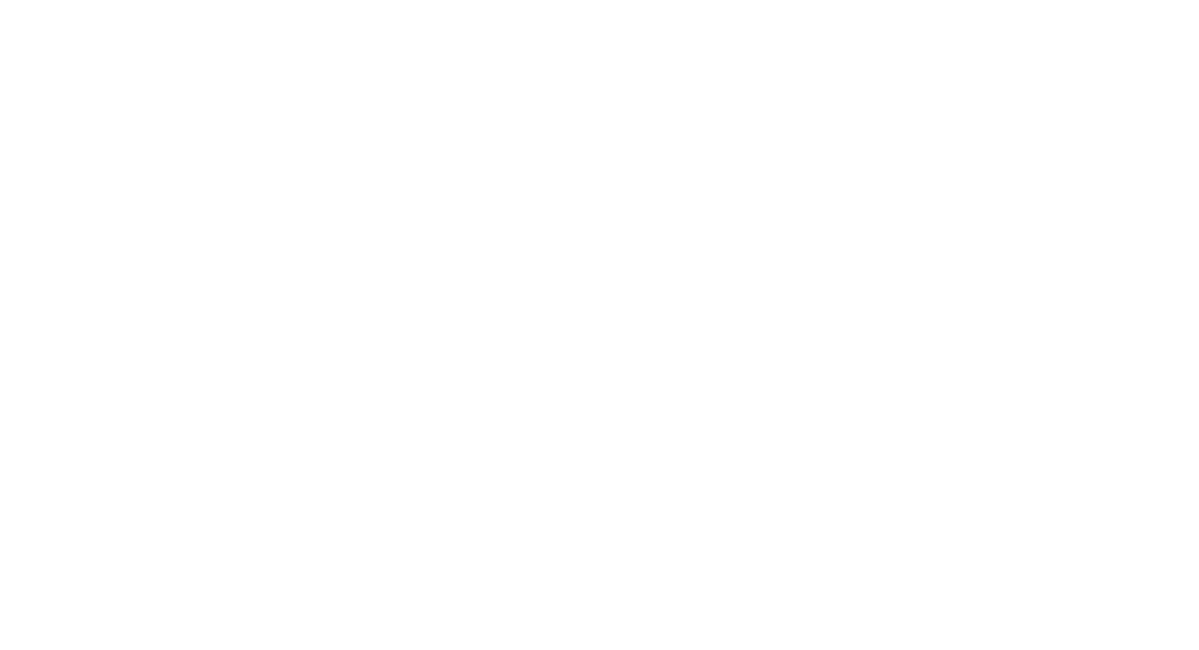The complexity to heapify an array is O(n log n) because it will traverse all the node [O(N)] and sift down each one of them [O(log n)].

A heapify behavior implemented in Go looks like this:

``````func Heapify(items []int) *BinaryHeap {
// create the binary heap
h := &BinaryHeap{items: items}

// lock meanwhile the operation is ongoing
h.mu.Lock()
defer h.mu.Unlock()

// sift down all the times starting from the last one
for idx := len(items) - 1; idx >= 0; idx-- {
h.siftDown(idx)
}

return h
}

func (h *BinaryHeap) siftDown(idx int) {
for {
// get the right and left index from the current one
switch rIdx, lIdx := h.right(idx), h.left(idx); {

// in case the right node doesn't exist in the tree
case rIdx >= len(h.items):
// if the left node exists and is greater than the current node swap it
if lIdx < len(h.items) && h.items[lIdx] > h.items[idx] {
h.items[idx], h.items[lIdx] = h.items[lIdx], h.items[idx]
}
// stop because the current node has smaller children
return

// in case the left node doesn't exist in the tree
case lIdx >= len(h.items):
// if the right node exists and is greater than the current node swap it
if rIdx < len(h.items) && h.items[rIdx] > h.items[idx] {
h.items[idx], h.items[rIdx] = h.items[rIdx], h.items[idx]
}
// stop because the current node has smaller children
return

// in case the current node is greater than both left and right nodes
// stop because the binary heap is sorted
case h.items[idx] > h.items[rIdx] && h.items[idx] > h.items[lIdx]:
return

// in case the left is greater than the right one
case h.items[rIdx] < h.items[lIdx]:
// swap the left with the current node
// update the index to the left index and continue sorting
h.items[idx], h.items[lIdx] = h.items[lIdx], h.items[idx]
idx = lIdx

// in case the right if greater than the left one
case h.items[rIdx] >= h.items[lIdx]:
// swap the right with the current node
// update the index to the right index and continue sorting
h.items[idx], h.items[rIdx] = h.items[rIdx], h.items[idx]
idx = rIdx

// in case none matched stop
default:
return
}
}
}

func (h *BinaryHeap) left(i int) int {
return i*2 + 1
}

func (h *BinaryHeap) right(i int) int {
return i*2 + 2
}
``````

## A min heap implementation#

To implement a min heap instead of a max heap, simply change the node value comparison from `<` to `>` and vice-versa in the siftUp and siftDown methods.

# Conclusion#

The binary heap is a really powerful data structure.

It has a lot of usages in real-life applications such as finding the shortest path (Dijkstra’s algorithm), implementing a heap sort algorithm, and operations where the higher the priority an element has, the sooner it will be extracted.

To end the article, here is a recap of the time complexity of some operations a binary heap can support:

Operation Time complexity
Extract O(log n)
Insert O(log n)
Heapify O(n log n)